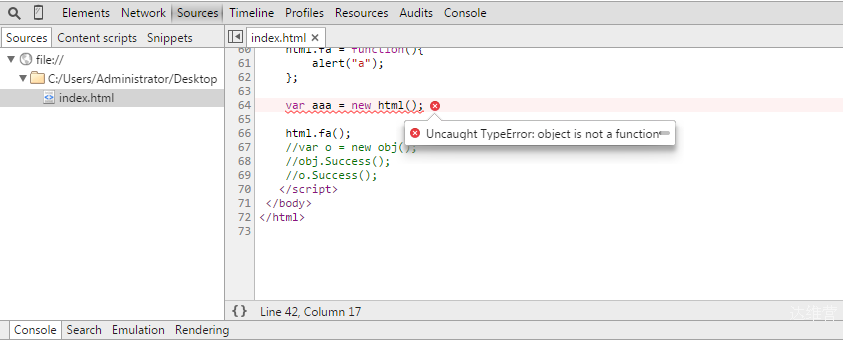﻿ Javascript闭包深入解析及实现方法-达维营-前端网

# Javascript闭包深入解析及实现方法

## 一、什么是闭包和闭包的几种写法和用法

1、什么是闭包

1. 作为一个函数变量的一个引用，当函数返回时，其处于激活状态。
2. 一个闭包就是当一个函数返回时，一个没有释放资源的栈区。

2、闭包的几种写法和用法

```//第1种写法
function Circle(r) {
this.r = r;
}
Circle.PI = 3.14159;
Circle.prototype.area = function() {
return Circle.PI * this.r * this.r;
}

var c = new Circle(1.0);

```//第2种写法
var Circle = function() {
var obj = new Object();
obj.PI = 3.14159;

obj.area = function( r ) {
return this.PI * r * r;
}
return obj;
}

var c = new Circle();

```//第3种写法
var Circle = new Object();
Circle.PI = 3.14159;
Circle.Area = function( r ) {
return this.PI * r * r;
}

```//第4种写法
var Circle={
"PI":3.14159,
"area":function(r){
return this.PI * r * r;
}
};

```//第5种写法
var Circle = new Function("this.PI = 3.14159;this.area = function( r ) {return r*r*this.PI;}");

```var dom = function(){

};

dom.Show = function(){
};

dom.prototype.Display = function(){
};

dom.Display(); //error
dom.Show();
var d = new dom();
d.Display();
d.Show(); //error```

1、不使用prototype属性定义的对象方法，是静态方法，只能直接用类名进行调用！另外，此静态方法中无法使用this变量来调用对象其他的属性！
2、使用prototype属性定义的对象方法，是非静态方法，只有在实例化后才能使用！其方法内部可以this来引用对象自身中的其他属性！

```var dom = function(){
var Name = "Default";
this.Sex = "Boy";
this.success = function(){
};
};

```var html = {
Name:'Object',
Success:function(){
this.Say = function(){
};
}
};```

```    var html = new Object();
html.Name = 'Object';
html.Success = function() {
this.Say = function () {
};
}``````alert(html.Name);
html.Success();```

```var s = new html.Success();
s.Say();

//还可以写到外面
html.Success.prototype.Show = function(){
};
var s = new html.Success();
s.Show();```

## 二、Javascript闭包的用途

1、匿名自执行函数

```var data= {
table : [],
tree : {}
};

(function(dm){
for(var i = 0; i < dm.table.rows; i++){
var row = dm.table.rows[i];
for(var j = 0; j < row.cells; i++){
drawCell(i, j);
}
}

})(data);```

2、结果缓存

```var CachedSearchBox = (function(){
var cache = {},
count = [];
return {
attachSearchBox : function(dsid){
if(dsid in cache){//如果结果在缓存中
return cache[dsid];//直接返回缓存中的对象
}
var fsb = new uikit.webctrl.SearchBox(dsid);//新建
cache[dsid] = fsb;//更新缓存
if(count.length > 100){//保正缓存的大小<=100
delete cache[count.shift()];
}
return fsb;
},

clearSearchBox : function(dsid){
if(dsid in cache){
cache[dsid].clearSelection();
}
}
};
})();

CachedSearchBox.attachSearchBox("input");```

3、封装

```var person = function(){
//变量作用域为函数内部，外部无法访问
var name = "default";

return {
getName : function(){
return name;
},
setName : function(newName){
name = newName;
}
}
}();

print(person.name);//直接访问，结果为undefined
print(person.getName());
person.setName("abruzzi");
print(person.getName());

undefined
default
abruzzi```

4、实现类和继承

```function Person(){
var name = "default";

return {
getName : function(){
return name;
},
setName : function(newName){
name = newName;
}
}
};

var p = new Person();
p.setName("Tom");
var Jack = function(){};
//继承自Person
Jack.prototype = new Person();
//添加私有方法
Jack.prototype.Say = function(){
};
var j = new Jack();
j.setName("Jack");
j.Say();

• QQ咨询
• 回顶